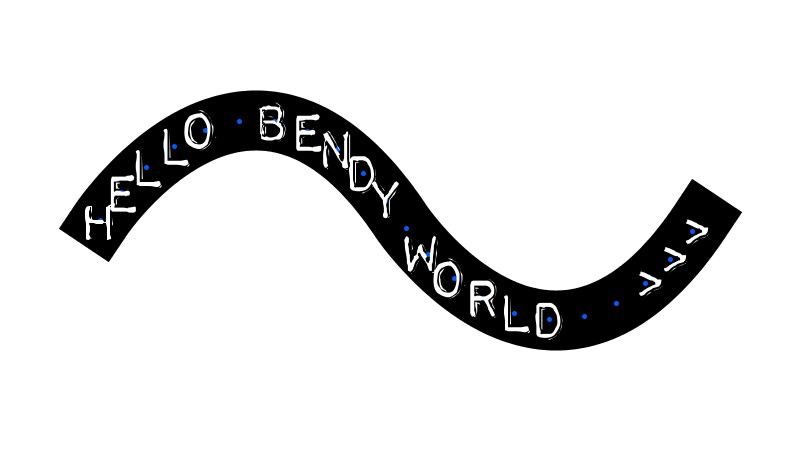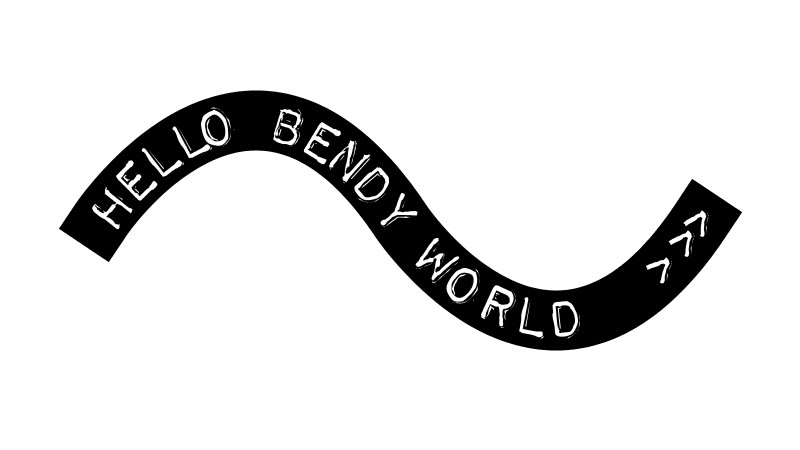# Placing text along a path using Geomerative and Processing

/, Programming, Projects, Uncategorized/Placing text along a path using Geomerative and Processing

## Introduction

In the last post we explored placing points along an a wave path we had created all using the built in functionality of the Geomerative library. This was ultimately to allow text to be placed along the path, which is what today’s post will cover.

If you have not read the previous posts, you will require a great library for processing named “Geomerative”  (Website) by Ricard Marxer.  I have struggled to find many similar sketches online other than those included in the examples, so i figured I would share my progress with the community which has always given me so much! This post whilst keeping a log for my own future use, I hope may serve as a reference for others out there with the same aims as me.

## First – Get some characters in place

So first up, lets just get some text on the points we established in the last post. This looks like:The code to make this:

```import geomerative.*;
RShape wave;
RFont font;

float plusminus =0.0;
float scale = 3;
String message = "hello bendy world &amp;gt;&amp;gt;&amp;gt;";
int index = 0;

//Somwhere to store our curve points
RPoint[] points;

void setup()
{
// From the examples we must always initialise the library using this command
RG.init(this);

//Usual Processing Jazz
size(800 ,450);
background(255);
frameRate(25);

font = new RFont("Impact Label Reversed.ttf", 72, RIGHT);
}

void draw(){
//Blank Background each frame
background(255);

//Create a new RShape each frame
RShape wave = new RShape();

//At the moment the wave object is empty, so lets add a curve:
wave.addBezierTo(0*scale, 100*scale, 50*scale, 25*scale, 100*scale, 100*scale);
wave.addBezierTo(100*scale, 100*scale, 150*scale, 175*scale, 200*scale, 100*scale);

//Lets draw away from the edges
translate(100,-80);

//Draw our wave
noFill();
stroke(0);
strokeWeight(60);
strokeCap(PROJECT);
wave.draw();
strokeCap(ROUND);

//Collect some points along the curve
RG.setPolygonizer(RG.UNIFORMLENGTH);
RG.setPolygonizerLength(35);
points = wave.getPoints();

index=0; //Letter index within the string message

//Loop through and place a letter at each point
for(int i=0; i&amp;lt;points.length-1; i++){
//Add a dot for the point
stroke(0, 90, 255); //Blue
strokeWeight(5);
point(points[i].x, points[i].y);

fill(255);
noStroke();

//Draw the current letter
pushMatrix();
translate(points[i].x, points[i].y);
//This is for centering up the font on the line
translate(5, 20);
font.draw(message.charAt(index));

popMatrix();

index++;
}
}
}
```

Now, this is a good first step, but we want our lettering to follow the curves, so next we must calculate the angle of each section of curve to correctly rotate our characters.

We can do this with a simple function calculating the angle between two points. Mine simply looks something like this:

```//Simple function to calculate the angle between two points
float getAngle(RPoint p1, RPoint p2){
float deltax = p1.x - p2.x;
float deltay= p1.y - p2.y;

return atan(deltay/deltax);
}
```

Now we can rotate the canvas where we were translating with a quick call to this new function:

```...
pushMatrix();
translate(points[i].x,points[i].y);
rotate(getAngle(points[i], points[i+1]));
translate(5, 20);
font.draw(message.charAt(index));
popMatrix();
...
```

Most definitely a step in the right direction, but we still see this is not perfect, because the text character is being placed on the first point, where as the angle is being calculated between the points. The best solution would be to place the characters in the center of the two points. We can do this with some simple calculation, or better still use the built in features of the Geomerative library! So after some exploring in the Geomerative Documentation I came across the getCenter() function, perfect!

``` stroke(255, 90, 0); //Orange
strokeWeight(10);
RPoint center = new RCommand(points[i], points[i+1]).getCenter();
point(center.x,center.y);
```

Turning off the text, we are not getting:

Now we place the characters in these new center points (center.x, center.y) and use the already calculated rotation resulting in:Complete code PDE can be found here:

https://github.com/louisc/processing-standalone-sketches/blob/master/Geomerative_Text_Along_Path/Geomerative_Text_Along_Path.pde

In Repo:

https://github.com/louisc/processing-standalone-sketches

By |2016-11-05T11:17:47+00:00June 28th, 2016|Kinetic Storyteller, Programming, Projects, Uncategorized|1 Comment1.